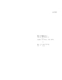## Multi-Dimensional Stochastic Ordering and Associated Random Variables.

 dc.contributor.author Baccelli, Francois en_US dc.contributor.author Makowski, Armand M. en_US dc.contributor.department ISR en_US dc.date.accessioned 2007-05-23T09:36:20Z dc.date.available 2007-05-23T09:36:20Z dc.date.issued 1987 en_US dc.description.abstract This paper presents several relationships between the notion of associated random variables and notions of stochastic ordering which have appeared in the literature over the years. More concretely, the discussion centers around the following question: Under which conditions does the association of the R-valued RV's {X_1, ... ,X_n} imply a possible ordering in some stochastic sense between the R^n-valued RV X := (X_1, ... ,X_n) and its independent version X := ({X PRIME_1}, ... , {X PRIME_n})? Some of the results in that direction are as follows: (i) These R^n- valued RV's are comparable in either one of the orderings <=_st, <=_ci, and <=_cv iff they are identical in law, and (ii) if the RV's {X_1, ... ,X_n} are associated, certain comparison properties hold for the stochastic orderings <=_D, <=_K, and <=_L, defined in Stoyan [8, p.27]. Strengthening of result (i) leads to the following results on the stochastic ordering properties of R^n-valued RV's X and Y with identical mean: (j) The RV's X and Y are comparable for <=_st iff they are identical in law, and (jj) If X <=_D Y (resP. X <=_K Y), then X and Y are comparable for <=_ci, (resp. <=_cv) iff they are identical in law. These and related results are given direct applications to queueing theory and to the asymptotics of associated random variables. en_US dc.format.extent 725693 bytes dc.format.mimetype application/pdf dc.identifier.uri http://hdl.handle.net/1903/4516 dc.language.iso en_US en_US dc.relation.ispartofseries ISR; TR 1987-7 en_US dc.title Multi-Dimensional Stochastic Ordering and Associated Random Variables. en_US dc.type Technical Report en_US
##### Original bundle
Now showing 1 - 1 of 1